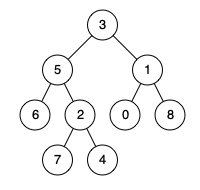# Leetcode 236 - LCA of a binary tree

Note:

• Base: root is null, p, or r, just return it.
• Do recursion on both left and right subtree.
• If we find both left and right, it means the current root is LCA.
• IF we only find either left or right, it means they are in the same subtree, and what the recursion returns is the LCA.

Given a binary tree, find the lowest common ancestor (LCA) of two given nodes in the tree.

According to the definition of LCA on Wikipedia: “The lowest common ancestor is defined between two nodes p and q as the lowest node in T that has both p and q as descendants (where we allow a node to be a descendant of itself).”

Example